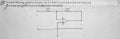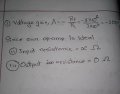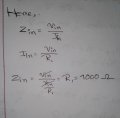# Op-amp input resistance and output resistance

#### studyhard24

Joined Jun 20, 2022
35My approach: (updated the gain value)As the question said the Op-amp is Ideal so I thought this could be the approach. But there are 5 marks for this question, so there must be something more to do here.
Let me know where I've mistaken, thank you for your precious time.

Last edited:

#### ericgibbs

Joined Jan 29, 2010
16,002

#### dl324

Joined Mar 30, 2015
14,902
As the question said the Op-amp is Ideal so I thought this could be the approach.
Your answers for impedance would only be correct if the opamp was the only component.

Check your math for the gain calculation.

#### dl324

Joined Mar 30, 2015
14,902
so the input resistance is
This is homework. You should delete your post.

#### studyhard24

Joined Jun 20, 2022
35
t
hi 24,
10^6 / 10^3 = ????

E
the other 0 is a bit far, Sorry for the inconvenience, Sir. The voltage gain calculation is easy. And as Mr Bob said the input resistance will be considered the 1K Ohm. I've understood that now, But what about the output resistance?? will it be the reference voltage i.e. 1 M ohms?

#### dl324

Joined Mar 30, 2015
14,902
as Mr Bob said the input resistance will be considered the 1K Ohm.
It's unfortunate that you were able to read his post before it could be removed. That isn't the way homework help is supposed to work. We're supposed to give hints to make you remember what you were taught. Giving you answers won't help you on tests and most schools would consider getting answers from others to be cheating.

#### ericgibbs

Joined Jan 29, 2010
16,002
will it be the reference voltage i.e. 1 M ohms?
hi 24,
Hint:
The OPA output resistance/impedance is internal, so what effect would that have if the impedance was 1meg.???

E

#### studyhard24

Joined Jun 20, 2022
35
It's unfortunate that you were able to read his post before it could be removed. That isn't the way homework help is supposed to work. We're supposed to give hints to make you remember what you were taught. Giving you answers won't help you on tests and most schools would consider getting answers from others to be cheating.
Sir, I have calculated the input impedance.#### crutschow

Joined Mar 14, 2008
30,438
But what about the output resistance?? will it be the reference voltage i.e. 1 M ohms?
Don't guess.
The output sees the 1Mohm feedback resistor in parallel with the op amp output.
So logically, what do you think would determine the output impedance?

#### BobTPH

Joined Jun 5, 2013
5,467
How does the output voltage change with the load? That would tell you the output impedance.

•crutschow

#### crutschow

Joined Mar 14, 2008
30,438
But there is no data sheet that was supposed to contain Zout, Aol.
The example op amp is ideal.
What is the gain and output impedance of an ideal op amp?

#### LvW

Joined Jun 13, 2013
1,478
Sir, the 1 M Ohm and Op-amp are parallel to each other.

View attachment 273253
But there is no data sheet that was supposed to contain Zout, Aol.

Like for this example:
View attachment 273254
Your formula for Zout contains the feedback effect (reduction factor due to loop gain) - and this is totally correct.
As a result, the output impedance is so small that in most cases it can be neglected - even without knowing some values for Aol and Rout (for the opamp alone)

#### studyhard24

Joined Jun 20, 2022
35
I get it now, the Output resistance of the circuit equals the output resistance of the OP-AMP i.e., zero ohms.

#### LvW

Joined Jun 13, 2013
1,478
I get it now, the Output resistance of the circuit equals the output resistance of the OP-AMP i.e., zero ohms.
No - consult your own result in post#11.
The output resistance of the opamp normally is app. (20...100) Ohms.
However, due to negative feedback the output resistance of the whole circuit is less by a factor (1+loop gain).
THAT is the reason you very often can neglect this value (because it is very small but never zero).

#### ericgibbs

Joined Jan 29, 2010
16,002
I get it now, the Output resistance of the circuit equals the output resistance of the OP-AMP i.e., zero ohms.
hi @studyhard24
In the original question the OPA is stated as Ideal, so your answer to this question, saying the output resistance is Zero ohms, is correct.E

#### LvW

Joined Jun 13, 2013
1,478
hi @studyhard24
In the original question the OPA is stated as Ideal, so your answer to this question, saying the output resistance is Zero ohms, is correct.E
Yes, thats correct as far as the original question (idealized opamp model) is concerned.
However, for my understanding we are in this discussion already one step further (see the contribution of studyhard24 in post '11). That is the background for my contribution in post'16 (real opamp).